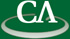Hybrid symbolic-numeric multiple integration and other applications of tensor product series

Keith Geddes, Symbolic Computation Group, University of Waterloo.

Wednesday November 1st, 10:30am in IRMACS 10908.

```Abstract:
A hybrid symbolic-numeric method for the fast and accurate evaluation of
definite integrals in multiple dimensions has been developed based on
approximating the multivariate integrand by tensor product series.
This method is well-suited for two classes of problems: (1) analytic
integrands over general regions in two dimensions, and (2) families of
analytic integrands with special algebraic structure over hyperrectangular
regions in higher dimensions.
Current numerical multiple integration methods either become very slow or
yield only low accuracy in high dimensions, due to the necessity to sample
the integrand at a very large number of points. Our approach overcomes this
difficulty by using a tensor product series with a modest number of terms
to construct an accurate approximation of the integrand. The partial
separation of variables achieved in this way reduces the original integral
to a manageable bilinear combination of integrals of half the original
dimension. We continue halving the dimensions recursively until obtaining
one-dimensional integrals, which are then computed by standard numeric or
symbolic techniques.

We have also applied tensor product series expansions as a technique for
deriving and proving identities for bivariate functions, as presented at
the Calculemus 2006 conference. Whereas our method for multidimensional
integration is based on approximating the integrand by a tensor product
series, the Calculemus paper exploits the concept of expanding a bivariate
function in a tensor product series, specifically focusing on cases where
the series terminates yielding a tensor product identity. The result is
an algorithm for the automatic derivation and proof of identities such as
the formula for cos(x+y) and similar identities for various elementary and
special functions.

This is Joint work with Frederick Chapman.
```